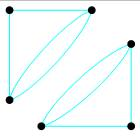# Determine (a) the number of edges in the graph, (b) the number of vertices in the graph, (c) the number of vertices that are of odd degree, (d) whether the graph is connected, and (e) whether the graph is a complete graph.### Mathematical Excursions (MindTap C...

4th Edition
Richard N. Aufmann + 3 others
Publisher: Cengage Learning
ISBN: 9781305965584

#### Solutions

Chapter
Section### Mathematical Excursions (MindTap C...

4th Edition
Richard N. Aufmann + 3 others
Publisher: Cengage Learning
ISBN: 9781305965584
Chapter 5.1, Problem 6ES
Textbook Problem
1 views

## Determine (a) the number of edges in the graph, (b) the number of vertices in the graph, (c) the number of vertices that are of odd degree, (d) whether the graph is connected, and (e) whether the graph is a complete graph.To determine

(a)

To determine the number of edges in the graph.

### Explanation of Solution

Given information:

Given graph is

To determine

(b)

To determine the number of vertices in the graph.

To determine

(c)

To determine the number of vertices that are of odd degree.

To determine

(d)

To determine whether the graph is connected.

To determine

(e)

To determine whether the graph is a complete graph.

### Still sussing out bartleby?

Check out a sample textbook solution.

See a sample solution

#### The Solution to Your Study Problems

Bartleby provides explanations to thousands of textbook problems written by our experts, many with advanced degrees!

Get Started

Find more solutions based on key concepts
In problems 24-26, find the intercepts and graph. 24.

Mathematical Applications for the Management, Life, and Social Sciences

In Exercises 14, find the values of x that satisfy the inequality (inequalities). 1. x + 3 2x + 9

Applied Calculus for the Managerial, Life, and Social Sciences: A Brief Approach

What is the value of (X + 1) for the following scores: 0, 1, 4, 2? a. 8 b. 9 c. 11 d. 16

Essentials of Statistics for The Behavioral Sciences (MindTap Course List)

Simplify the expressions in Exercises 97106. x1/2yx2y3/2

Finite Mathematics and Applied Calculus (MindTap Course List)

The area of the region bounded by , , and r = sec θ is:

Study Guide for Stewart's Multivariable Calculus, 8th

Which point x1, x2, x3, or x4, on the graph of y = f(x) is the best choice to serve as the point guaranteed by ...

Study Guide for Stewart's Single Variable Calculus: Early Transcendentals, 8th

In Problems 2734 find linearly independent functions that annihilated by the given differential operator. 32. D...

A First Course in Differential Equations with Modeling Applications (MindTap Course List)

52. Advertisers contract with Internet service providers and search engines to place ads on websites. They pay ...

Modern Business Statistics with Microsoft Office Excel (with XLSTAT Education Edition Printed Access Card) (MindTap Course List)

Evaluating Formulas In Exercises S-1 through S-24, evaluate the given function as required. Calculate A(5.1,3.2...

Functions and Change: A Modeling Approach to College Algebra (MindTap Course List)